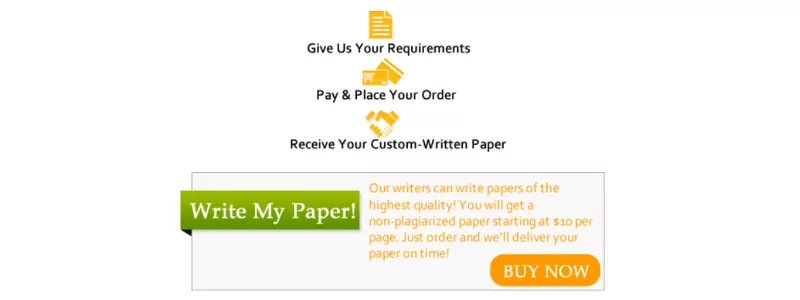# Barbara Bright is the purchasing agent for West Valve Company

1. Barbara Bright is the purchasing agent for West Valve Company. West Valve sells industrial valves and fluid control devices. One of the most popular valves is the Western, which has an annual demand of 4,000 units. The cost of each valve is \$90, and the inventory carrying cost is estimated to be 10% the cost of each valve. Barbara has made a study of the costs involved in placing an order for any of the valves that West Valve stocks, and she has concluded that the average ordering cost is \$25 per order. Furthermore, it takes about two weeks for an order to arrive from the supplier, and during this time the demand per week for West valves is approximately 80.

A)    what is the EOQ

B)    What is the ROP

C)    What is the average inventory? What is the annual holding cost?

D) How many orders per year would be placed? What is the annual ordering cost?

2. Ross White’s machine shop uses 2,500 brackets during the course of a year, and this usage is relatively constant throughout the year. These brackets are purchased from a supplier a 100 miles away for \$15 each, and the lead time is 2 days. The holding cost per bracket per year is \$1.50 (or 10% of the unit cost) and the ordering cost per order is \$18.75. There are 250 working days per year.

A)    What is the EOQ

B)    Given the EOQ, what is the average inventory? What is the annual inventory holding cost?

C)    In minimizing cost, how many orders would be made each year? What would be the annual ordering cost?

D)    Given the EOQ, what is the total annual inventory cost (cost including purchase cost)

E)    What is the time between orders?

F) What is the ROP?

3. Linda Lechner is in charge of maintaining hospital supplies at General Hospital. During the past year, the mean lead time demand for bandage BX-5 was 60. Furthermore, the standard deviation for BX-5 was 7. Linda would like to maintain a 90% service level. What safety stock level do you recommend for BX-5?

4. Shoe Shine is a local retail shoe located on the north side of Centerville. Annual demand for a popular sandal is 500 pairs, and John Dirk, the owner of Shoe Shine, has been in the habit of ordering 100 pairs at a time. John estimates that the ordering cost is \$10 per order. The cost of the sandal is \$5 per pair. For John’s ordering policy to be correct, what would the carrying cost as a percentage of the unit cost have to be? If the carrying cost were 10% of the cost, what would the optimal order quantity be?

Is this the question you were looking for? If so, place your order here to get started!